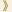HomeShaw Laureates2019Mathematical SciencesPress Release

The Shaw Prize in Mathematical Sciences 2019 is awarded to Michel Talagrand, Professor of Mathematics at the Sorbornne University, France, for his work on concentration inequalities, on suprema of stochastic processes and on rigorous results for spin glasses.

Michel Talagrand has made profound contributions to probability and high-dimensional geometry, at least three of which could be described as revolutionary.

A first major theme of Talagrand’s research is the study of suprema of stochastic processes. A stochastic process is a collection of interacting random variables. When one is given a large such collection, it is often of crucial importance to obtain information about how its maximum value is distributed. Starting with the case of Gaussian processes (here the random variables each have a Gaussian distribution, given by the famous “bell curve”, and can be correlated in a certain way) and then for more general cases, Talagrand has developed tools, such as majorizing measures or generic chaining, that provide powerful and very useful bounds for how these maximum values behave.

The second group of contributions concerns a phenomenon known as concentration of measure. Broadly speaking, this says that many functions that depend on a large number of reasonably independent random variables are extremely likely to take values close to their average. For example, if one tosses a coin a thousand times, then the probability that the number of heads will be between 450 and 550 is roughly 99.7 percent, and the probability that it will be more than 600 is approximately two millionths of one percent. In such a situation, we say that the number of heads is concentrated. This phenomenon, often associated with the name of the mathematician Vitali Milman, is remarkably general and has a multitude of applications in areas as diverse as the geometry of convex bodies, graph theory, and theoretical computer science. One of Talagrand's great achievements has been to examine this phenomenon in detail and hugely improve our understanding of it. In particular, he proved famous inequalities, using completely new techniques, that give new concentration results that are widely used in many different important settings.

A third family of results for which he is famous concern objects known as spin glasses, which provide a mathematical model of a physical phenomenon involving very disordered systems. Unlike many models from statistical physics, spin glasses have a double layer of randomness. First, the way in which different random variables (the spins in the spin glass language) will interact is chosen at random, which creates a very complex energy landscape, and then the random variables themselves are sampled randomly. One would then like to understand this large family of randomly interacting random variables and describe its typical features. Spin glasses have a short and simple definition, but they are notoriously hard to analyze. A significant advance was made by the theoretical physicist, Giorgio Parisi, who proposed a formula for the free energy of a spin glass, which is an important quantity that encapsulates information about this random energy landscape. However, turning predictions of statistical physicists into mathematically rigorous arguments is often extremely hard, and a rich source of fascinating mathematical problems. Finding a complete rigorous proof in this case seemed to be way beyond what was it was realistic to hope for, despite remarkable insights and progress by Francesco Guerra, but Talagrand managed to do it, thereby providing for the first time a complete mathematical underpinning for this extremely important physical theory.

One notable feature of Talagrand’s career that marks him out from many other mathematicians is that when he solves a problem, he does not just leave it and move on. Rather, he continues to work on it, improving his understanding and reworking his arguments until he has a well-developed theory that can be more easily used by other mathematicians. He has written monumental and highly influential textbooks on all the three topics just mentioned, and these have played a very significant part in the spread of his ideas, which are now central to the work of large numbers of other mathematicians. Talagrand is a true one-off, nearly always working on his own, and obtaining extraordinary and highly unexpected results that have changed the mathematical landscape.

Mathematical Sciences Selection Committee
The Shaw Prize

21 May 2019  Hong Kong